# Statistics¶

In this section we look at a list of numbers and calculate

• minimum value and its position
• maximum value and its position
• sum
• average

## Random list¶

For this exercice we use a list with 8 random numbers between 1 and 99. This way we can print them on the first line of the display.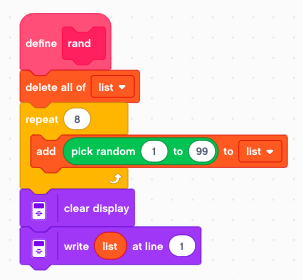We call this function in the start event and also with the left button.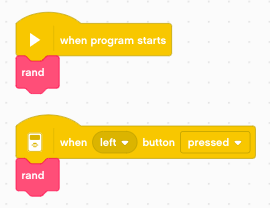The result of this function looks like this:

```86 35 49 54 37 6 93 62
```

## Calculate the minimum¶

At the first iteration i=1 we set

• min = list
• minpos = 1

Then we iterate through the rest of the list. If we find a number which is smaller, we take it as the new minimum.

• min = list[i]
• minpos = i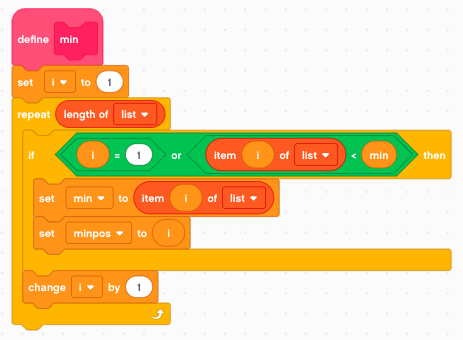We call this function with the down button.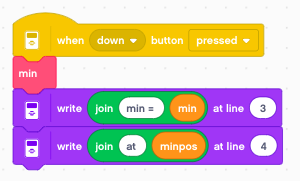The result of this function looks like this:

```86 35 49 54 37 6 93 62

min = 6
at 6
```

## Calculate the maximum¶

Again, at the first iteration i=1 we set

• max = list
• maxpos = 1

Then we iterate through the rest of the list. If we find a number which is larger, we take it as the new maximum.

• max = list[i]
• maxpos = i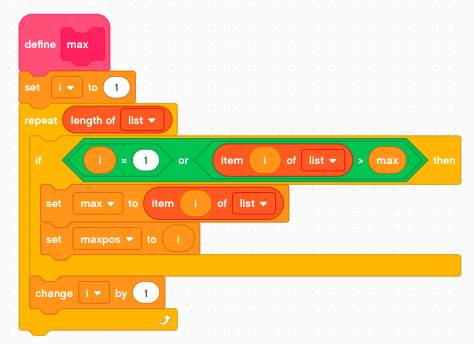We call this function with the up button.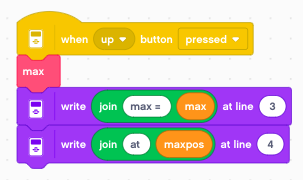The result of this function looks like this:

```86 35 49 54 37 6 93 62

max = 93
at 7
```

## Calculate sum and average¶

To get the sum we add all elements of the list together. The average is obtained by dividing the sum by the number of elements.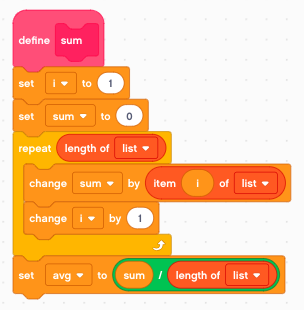We call this function with the center button.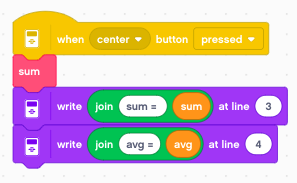The result of this function looks like this:

```86 35 49 54 37 6 93 62

max = 422
avg = 52.75
```